Definitions of Square Dance Calls and Concepts
Cross Invert The Column ({fraction}) [C2]

Index -->  Plus  |  A1  |  A2  |  C1  |  C2  |  C3A  |  C3B  |  C4  |  NOL  |
Definitions (Text Only) -->  Plus  |  A1  |  A2  |  C1  |  C2  |  C3A  |  C3B  |  C4  |  NOL  |
 Find call:

Cross Invert The Column (fraction) -- [C2]
(author unknown)

 \$B8@8l(B

From Parallel Columns of {n}, where n is greater than or equal to 3.

The First n dancers (where n corresponds to the given fraction, see first note below) as a unit Trail Off to end in Concentric Columns of n as the remaining dancers, using Circulates, move forward to form a compact formation.

\$BCm
• From Parallel Columns of 4, n=1 for 1/4; 2 for 1/2; 3 for 3/4; or 4 (full) if no fraction is given.
• From Parallel Columns of 3, n=1 for 1/3; 2 for 2/3; or 3 (full) if no fraction is given.
• On a full Cross Invert The Column, everyone does the Trail Off movement but must also slide together upon completion of the call to form adjacent Columns. Do not slide together if call is fractionalized (e.g., 3/4 Cross Invert The Column ends in an "H").
•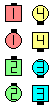Cross Invert The Column\$B\$NA0(B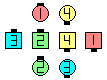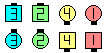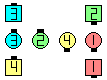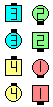1/4\$B\$N8e(B 1/2\$B\$N8e(B 3/4\$B\$N8e(B (\$B=*\$o\$j(B)

Variation:Magic Cross Invert The Column fraction [C2V].

\$BInvert The Column (fraction) [C2].Choreography for Cross Invert The Column (fraction)Comments? Questions? Suggestions?

https://www.ceder.net/def/crossinvertthecolumn.php?language=japan&level=C2
14-December-2019 08:11:37